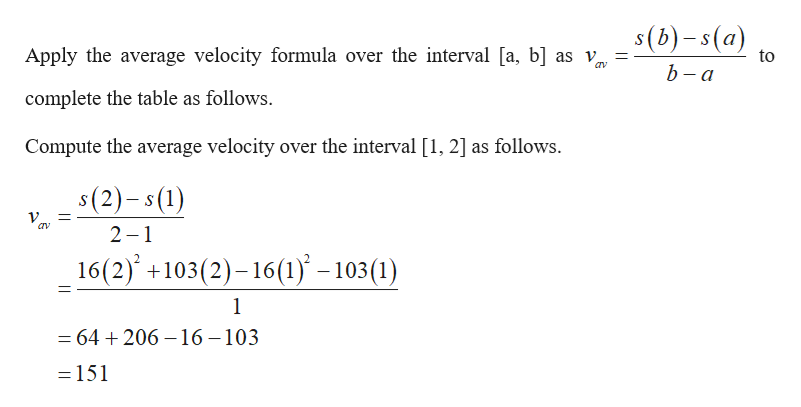For the position function, s(t)=-16t2+103t​, complete the following table with the appropriate average velocities. Then make a conjecture about the value of the instantaneous velocity at t​ = 1.time interval [1,2][1,1.5][1,1.1][1,1.01][1,1.001]average velocity

Question

For the position function, s(t)=-16t2+103t​, complete the following table with the appropriate average velocities. Then make a conjecture about the value of the instantaneous velocity at t​ = 1.

 time interval [1,2] [1,1.5] [1,1.1] [1,1.01] [1,1.001] average velocity
Step 1

Consider the position function, s(t) = 16t2+103t.help_outlineImage Transcriptioncloses(b)-s(a) Apply the average velocity formula over the interval [a, b] as v to b - a complete the table as follows. Compute the average velocity over the interval [1, 2] as follows. s(2)-s(1) 2 1 16(2) +103(2)-16(1 -103 (1) 1 = 64 206-16 -103 - 151 fullscreen
Step 2

Compute the average velocity over the...

Want to see the full answer?

See Solution

Want to see this answer and more?

Our solutions are written by experts, many with advanced degrees, and available 24/7

See Solution
Tagged in

Other